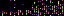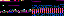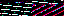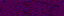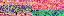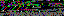```EVR.include("level/cluster/Beam.js");
EVR.Level.Cluster = function(passage, width, gap, practice)
{
this.passage = passage - 1;
this.beam_width = parseFloat(width);
this.gap = parseFloat(gap);
this.practice = practice;
this.dimensions = [0, 0];
}
EVR.Level.Cluster.prototype = new EVR.Graphic;
EVR.Level.Cluster.prototype.update_dimensions = function()
{
var remove = false;
if (!this.attached)
{
var remove = true;
this.append();
}
this.dimensions = EVR.Graphic.prototype.get_dimensions.call(this);
if (remove)
{
this.remove();
}
}
EVR.Level.Cluster.prototype.get_dimensions = function()
{
return this.dimensions;
}
EVR.Level.Cluster.prototype.initiate = function(container, level)
{
EVR.Graphic.call(
this, container, RATIO_HEIGHT, null, null, container.container);
this.beam_height = container.beam_height;
this.colors = level.beams;
this.margin = container.margin;
this.set_proportions();
this.populate();
this.update_dimensions();
}
EVR.Level.Cluster.prototype.set_proportions = function()
{
var width = this.calculate_width();
var height = this.container.get_dimensions(true);
EVR.Graphic.prototype.set_proportions.call(this, width, height);
}
EVR.Level.Cluster.prototype.calculate_width = function()
{
var sum = this.beam_width + this.gap;
var count = this.colors.length;
var divisor = count + (this.margin / this.beam_height) * (count - 1);
return sum / divisor;
}
EVR.Level.Cluster.prototype.populate = function()
{
this.beams = [];
for (var ii = 0; ii < this.colors.length; ii++)
{
this.beams.push(new EVR.Level.Cluster.Beam(this, ii, this.practice));
}
}
EVR.Level.Cluster.prototype.draw = function()
{
EVR.Graphic.prototype.draw.call(this);
if (!!this.beams)
{
for (var ii = 0; ii < this.beams.length; ii++)
{
this.beams[ii].draw();
}
}
this.update_dimensions();
}
EVR.Level.Cluster.prototype.get_gap_x = function()
{
var x = this.get_coordinates();
var width = this.beams.get_dimensions();
return x + width;
}
EVR.Level.Cluster.prototype.toString = function()
{
return "[object EVR.Level.Cluster]";
}
```
```EVR.Level.Cluster.Beam = function(container, index, practice)
{
this.container = container;
this.index = index;
this.practice = practice;
this.width = container.beam_width;
this.height = container.beam_height;
this.color = container.colors[index];
this.dimensions = [0, 0];
this.construct();
}
EVR.Level.Cluster.Beam.prototype = new EVR.Beam;
EVR.Level.Cluster.Beam.prototype.construct = function()
{
var relative = this.container.size_relative;
var color = this.color;
var border = BEAM_BORDER;
if (this.practice && this.index == this.container.passage)
{
color = null;
border = BEAM_PASSAGE_BORDER;
}
EVR.Beam.call(
this, this.container, color, this.width, this.height, null, null,
relative);
this.css.border = border;
this.orient();
}
EVR.Level.Cluster.Beam.prototype.shape = function()
{
EVR.Beam.prototype.shape.call(this);
this.update_dimensions();
if (!!!window.ActiveXObject)
{
var dimensions = this.get_dimensions();
this.set_dimensions(dimensions - 4, dimensions - 4);
this.update_dimensions();
}
}
EVR.Level.Cluster.Beam.prototype.orient = function()
{
var container = this.container;
var margin = container.margin;
var height = container.beam_height;
var offset = height + margin;
this.place(null, offset * this.index);
}
EVR.Level.Cluster.Beam.prototype.update_dimensions = function()
{
this.dimensions = EVR.Graphic.prototype.get_dimensions.call(this);
}
EVR.Level.Cluster.Beam.prototype.get_dimensions = function()
{
return this.dimensions;
}
EVR.Level.Cluster.Beam.prototype.toString = function()
{
return "[object EVR.Level.Cluster.Beam]";
}
```
```EVR.include("level/countdown/Glyph.js");
EVR.Level.Countdown = function(level, container, action)
{
this.level = level;
this.container = container;
this.action = action;
this.length = COUNTDOWN_LENGTH;
this.delay = COUNTDOWN_DELAY;
this.rate = 50;
this.elapsed = new EVR.Time();
this.build();
}
EVR.Level.Countdown.prototype.build = function()
{
this.glyphs = [];
for (var ii = this.length; ii > 0; ii--)
{
this.glyphs.push(new EVR.Level.Countdown.Glyph(this.container, ii));
}
}
EVR.Level.Countdown.prototype.start = function()
{
this.level.set_state(LEVEL_STATE_WAITING, false);
this.loop(this.delay);
}
EVR.Level.Countdown.prototype.update = function()
{
var difference = this.set_time();
var seconds = this.elapsed.get_seconds();
if (seconds < this.length)
{
if (typeof(this.seconds) == "undefined")
{
this.glyphs.append();
}
else if (seconds > this.seconds)
{
this.glyphs[seconds - 1].remove();
this.glyphs[seconds].append();
}
else
{
this.glyphs[seconds].grow(difference / 1000);
}
this.seconds = seconds;
this.loop(this.rate);
}
else
{
this.glyphs[seconds - 1].remove();
this.action();
}
}
EVR.Level.Countdown.prototype.set_time = function()
{
var difference = 0;
var time = +new Date;
if (this.time)
{
difference = time - this.time;
}
this.time = time;
return difference;
}
EVR.Level.Countdown.prototype.loop = function(delay)
{
var current = this;
window.setTimeout(
function()
{
current.update();
}, delay);
}
EVR.Level.Countdown.prototype.draw = function()
{
var glyph, glyphs = this.glyphs;
for (var ii = 0; ii < this.length; ii++)
{
glyph = glyphs[ii];
glyph.attached && glyph.draw();
}
}
EVR.Level.Countdown.prototype.toString = function()
{
return "[EVR.Level.Countdown]";
}
```
3.239.129.52
3.239.129.52
3.239.129.52

September 13, 2013

```from array import array
from time import sleep

import pygame
from pygame.mixer import Sound, get_init, pre_init

class Note(Sound):

def __init__(self, frequency, volume=.1):
self.frequency = frequency
Sound.__init__(self, self.build_samples())
self.set_volume(volume)

def build_samples(self):
period = int(round(get_init() / self.frequency))
samples = array("h",  * period)
amplitude = 2 ** (abs(get_init()) - 1) - 1
for time in xrange(period):
if time < period / 2:
samples[time] = amplitude
else:
samples[time] = -amplitude
return samples

if __name__ == "__main__":
pre_init(44100, -16, 1, 1024)
pygame.init()
Note(440).play(-1)
sleep(5)
```

This program generates and plays a 440 Hz tone for 5 seconds. It can be extended to generate the spectrum of notes with a frequency table or the frequency formula. Because the rewards in Send are idealized ocean waves, they can also be represented as tones. Each level has a tone in its goal and a tone based on where the player's disc lands. Both play at the end of a level, sounding harmonic for a close shot and discordant for a near miss. The game can dynamically create these tones using the program as a basis.

I'm also building an algorithmically generated song: Silk Routes (Scissored). Here is an example of how it sounds so far.